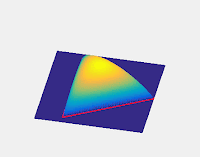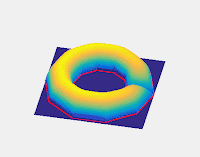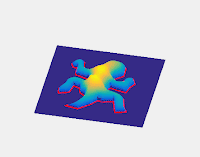## A Circle Theorem

Take a circle of radius $R$. Select a point $A$ inside it a distance $a$ from the center, with $a < R$. From $A$, construct $N>1$ line segments starting from A and touching the circle, segment k touching the circle at $P_k$, such that if $a-b\equiv \pm 1 \mod N$, then $\measuredangle P_aAP_b=2\pi/N$, that is all the segments are equally-angularly-spaced. Let $d_k=\overline{AP_k}$. Then $\prod_{k=1}^{N}d_k=\prod_{k=1}^{N}\left ( a\cos \left ( \theta_0+\frac{2 k \pi}{N} \right )+\sqrt{R^2-a^2+a^2\cos^2 \left ( \theta_0+\frac{2 k \pi}{N} \right )} \right ) \\ \prod_{k=1}^{N}d_k=\prod_{k=1}^{N}\left (\sqrt{R^2-a^2}\exp\left ( \sinh^{-1}\left (\frac{a}{\sqrt{R^2-a^2}}\sin \left ( \theta'_0+\frac{2 k \pi}{N} \right ) \right ) \right ) \right )$ Therefore $\sqrt[N]{\prod_{k=1}^{N}d_k}=\sqrt{R^2-a^2}\exp \left (\frac{1}{N}\sum_{k=1}^{N} \sinh^{-1}\left (\frac{a}{\sqrt{R^2-a^2}}\sin \left ( \theta'_0+\frac{2 k \pi}{N} \right ) \right ) \right )$ It follows that, for N even, as the summation will cancel in every term, $\sqrt[N]{\prod_{k=1}^{N}d_k}=\sqrt{R^2-a^2}$ This also holds asymptotically, as the error approaches zero. it is generally not true for N odd.

Note that this much more widely generalizes the well-known geometric mean theorem. This can be seen as a consequence of the power of a point theorem.

A pleasant interpretation of this is that if we take a diametric cross-section of a sphere and choose a point on that disk, the height of the sphere above that point is the geometric mean of the legs of any $2N>1$ equiangular, planar, stellar net connecting that point to the boundary of the disk.

## A Related Volumizing Algorithm

This theorem suggests an algorithm for producing a 3D volume given a closed 2D boundary shape. If we assume the 2D shape is of a diametric cross-section, we simply apply the method detailed above to produce the height above that point. That is, for a given point inside the shape, we take an N-leg equiangular stellar net emanating from that point to the boundary of the shape. The height of the surface at that point is then the geometric mean of the N legs of that net.

This method ensures that circular shapes produce spherical surfaces. However, if N is low, for less regular boundary shapes, the resulting surface may be quite lumpy or sensitive to how the angles of each net are chosen. One solution, then, is simply to make N large enough. However, this may end up being computationally expensive.

In theory, it may be possible to find the asymptotic value: find all parts of the boundary shape visible from the given point, and find the integral of the log of the distance, sweeping over the angle. If the boundary is a polygon, this involves evaluating (or approximating) integrals of the form $\int\ln\left ( \sin(x) \right)dx$ Which have no general closed form in terms of elementary functions. However, we can evaluate certain cases. One easy example is that of an infinite corridor formed from two parallel lines. We find that the height profile is double that of a circular cylinder. It may be desirable, then, to determine another function to multiply by which will halve the heights of corridors but leave hemispheres undisturbed.

Below we give some visual examples of the results of the algorithm. The original 2D shapes are shown in red.In order, an equilateral triangle, an icosagon, a five-pointed star, an almost-donut, an Escherian tesselating lizard, and a tesselating spider.

## Sunday, February 18, 2018

### Rotating Fluid

Suppose we have an infinitely tall cylinder of radius R, filled to a height H with an incompressible fluid. We then set the fluid rotating about the cylindrical axis at angular speed $\omega$. Suppose we take a differential chunk of fluid on the surface, a radius r from the axis. The resulting normal force will then be $N=F_c+W$. This normal force, as the name suggests, will be normal to the fluid surface. It follows by simple geometry, that $\frac{dy}{dr}=\frac{F_c}{W}=\frac{r \omega^2}{g}$ From which it follows that the height of the surface at any radius will be given by $y=\frac{r^2 \omega^2}{2g}+C$ Let us define $\omega_0=2\sqrt{gH}/R \\ u=\omega/\omega_0$ Given that the fluid is incompressible, we know that the total volume does not change. From this, we can determine that the height of the surface at any radius will be given by: $y(r)=2H\left ( ru/R \right )^2+\left\{\begin{matrix} H(1-u^2) \\ 2H(u-u^2) \end{matrix}\right. \, \, \, \, \, \, \, \begin{matrix} u \leq 1\\ u > 1 \end{matrix}$ The highest point on the liquid surface is then given by: $y_{\textrm{max}}=\left\{\begin{matrix} H(1+u^2)\\ 2Hu \end{matrix}\right. \, \, \, \, \, \, \, \begin{matrix} u \leq 1\\ u > 1 \end{matrix}$ If $u > 1$, the center of the base of the cylinder is not covered by fluid. There is a minimum radius at which fluid can be found. This minimum radius is given by: $r_{\textrm{min}}=R\sqrt{1-\frac{1}{u}}$ If the fluid is of uniform density and of total mass M, then the moment of inertia of the rotating fluid is given by $I=\left\{\begin{matrix} \frac{MR^2}{2}\left ( 1+\frac{u^2}{3} \right )\\ MR^2\left ( 1-\frac{1}{3u} \right ) \end{matrix}\right. \, \, \, \, \, \, \, \begin{matrix} u \leq 1\\ u > 1 \end{matrix}$ Note for each of these piecewise functions, the functions and their first derivatives are continuous.

## Bias in Performance Evaluation

Suppose you are an employer. You are looking to fill a position and you want the best person for the job. To do this, you take a pool of applicants, and for each one, you test them N times on some metric X. From these N tests, you will develop some idea of what each applicant's performance will look like, and based on that, you will hire the applicant or applicants with the best probable performance. However, you know that each applicant comes from one of two populations which you believe to have different statistical characteristics, and you know immediately which population each applicant comes from.

We will use the following model: We will assume that the population from which the applicants are taken is made up of two sub-populations A and B. These two sub-populations have different distributions of individual mean performance that are both Gaussian. That is, an individual drawn from sub-population A will have an expected performance that is normally distributed with mean $\mu_A$ and variance $\sigma_A^2$. Likewise, an individual drawn from sub-population B will have an expected performance that is normally distributed with mean $\mu_B$ and variance $\sigma_B^2$. Individual performances are then taken to be normally distributed with the individual mean and individual variance $\sigma_i^2$.

Suppose we take a given applicant who we know comes from sub-population B. We sample her performance N times and get performances of $\{x_1,x_2,x_3,...,x_N\}=\textbf{x}$. We form the following complete pdf for the (N+1) variables of the individual mean and the N performances: $f_{\mu_i,\textbf{x}|B}(\mu_i,x_1,x_2,...,x_N)=\frac{1}{\sqrt{2\pi}^{N+1}}\frac{1}{\sigma_B \sigma_i^N} \exp\left ({-\frac{(\mu_i-\mu_B)^2}{2\sigma_B^2}} \right ) \prod_{k=1}^N\exp\left ({-\frac{(x_k-\mu_i)^2}{2\sigma_i^2}} \right )$ It follows that the distribution conditioned on the test results is proportional to: $f_{\mu_i|,\textbf{x},B}(\mu_i)\propto \exp\left ({-\frac{(\mu_i-\mu_B)^2}{2\sigma_B^2}} \right ) \prod_{k=1}^N\exp\left ({-\frac{(x_k-\mu_i)^2}{2\sigma_i^2}} \right )$ By normalizing we find that this implies that the individual mean, given that it comes from sub-population B and given the N test results, is normally distributed with variance $\sigma_{\tilde{\mu_i}}^2=\left ( {\frac{1}{\sigma_B^2}+\frac{N}{\sigma_i^2}} \right )^{-1}$ and mean $\tilde{\mu_i}=\frac{\frac{\mu_B}{\sigma_B^2}+\frac{1}{\sigma_i^2}\sum_{k=1}^{N}x_k}{\frac{1}{\sigma_B^2}+\frac{N}{\sigma_i^2}} =\frac{\frac{\mu_B}{\sigma_B^2}+\frac{N}{\sigma_i^2}\bar{\textbf{x}}}{\frac{1}{\sigma_B^2}+\frac{N}{\sigma_i^2}}$ We will assume that this mean and variance are used as estimators to predict performance. Note that, in the limit of large N, $\sigma_{\tilde{\mu_i}}^2\rightarrow \sigma_i^2/N$ and $\tilde{\mu_i}\rightarrow \bar{\textbf{x}}\rightarrow \mu_i$, as expected.

Suppose we assume sub-populations A and B have the same variance $\sigma_{AB}^2$, but $\mu_A>\mu_B$. then we can note the following few implications:
• The belief about the sub-population the applicant comes from acts effectively as another performance sample of weight $\sigma_i^2/\sigma_{AB}^2$.
• If applicant 1 comes from sub-population A and applicant 2 comes from sub-population B, even if they perform identically in their samples, applicant 1 would nevertheless still be preferred.
• The more samples are taken, the less the sub-population the applicant comes from matters.
• The larger the difference in means between the sub-populations is assumed to be, the better the lesser-viewed applicant will need to perform in order to be selected over the better-viewed applicant.
• Suppose we compare $\tilde{\mu_i}$ to $\bar{\textbf{x}}$. Our selection criteria will simply be if the performance predictor is above $x_m$. We want to find the probability of being from a given sub-population given that the applicant was selected by each predictor. For the sub-population-indifferent predictor: $P(A|\bar{\textbf{x}}\geq x_m)=\frac{P(\bar{\textbf{x}}\geq x_m|A)P(A)}{P(\bar{\textbf{x}}\geq x_m|A)P(A)+P(\bar{\textbf{x}}\geq x_m|B)P(B)} \\ \\ P(A|\bar{\textbf{x}}\geq x_m)= \frac{P(A)Q\left (\frac{x_m-\mu_A}{\sqrt{\sigma_{AB}^2+\sigma_i^2/N}} \right )} {P(A)Q\left (\frac{x_m-\mu_A}{\sqrt{\sigma_{AB}^2+\sigma_i^2/N}} \right ) + P(B)Q\left (\frac{x_m-\mu_B}{\sqrt{\sigma_{AB}^2+\sigma_i^2/N}} \right )}$ Where $Q(z)=\int_{z}^{\infty}\frac{e^{-s^2/2}}{\sqrt{2\pi}}ds\approx \frac{e^{-z^2/2}}{z\sqrt{2\pi}}$ For the sub-population-sensitive predictor, we first note that $\tilde{\mu_i} \geq x_m \Rightarrow \bar{\textbf{x}}\geq x_m+(x_m-\mu_A)\frac{\sigma_i^2}{N\sigma_A^2}=x_m'$ Which then implies $P(A|\tilde{\mu_i}\geq x_m)=\frac{P(\tilde{\mu_i}\geq x_m|A)P(A)}{P(\tilde{\mu_i}\geq x_m|A)P(A)+P(\tilde{\mu_i}\geq x_m|B)P(B)} \\ \\ P(A|\tilde{\mu_i}\geq x_m)= \frac{P(A)Q\left (\frac{x_m'-\mu_A}{\sqrt{\sigma_{AB}^2+\sigma_i^2/N}} \right )} {P(A)Q\left (\frac{x_m'-\mu_A}{\sqrt{\sigma_{AB}^2+\sigma_i^2/N}} \right ) + P(B)Q\left (\frac{x_m'-\mu_B}{\sqrt{\sigma_{AB}^2+\sigma_i^2/N}} \right )}$ As $x_m > \mu_A$ and thus $x_m' > x_m$, it is easy to see that $P(A) < P(A|\bar{\textbf{x}}\geq x_m) < P(A|\tilde{\mu_i}\geq x_m)$. Thus the sensitivity further biases the selection towards sub-population A. We can call $\bar{\textbf{x}}$ the meritocratic predictor and $\tilde{\mu_i}$ the semi-meritocratic predictor.

## Some Sociological Implications

Though the above effects may, in theory, be small, their effects in practice may not be. Humans are not perfectly rational and are not perfect statistical computers. The above is meant to give motivation for taking seriously effects that are often much more pronounced. If there is a perceived difference in means, there is likely a tendency to exaggerate it, to think that the difference in means should be visible, and hence that the two distributions should be statistically separable. Likewise, population variances are often perceived as narrower than they really are, leading to further amplification of the biasing effect. Moreover, the parameter estimations are not based simply on objective observation of the sub-populations, but also if not mainly on subjective, sociological, psychological, and cultural factors. As high confidence in one's initial estimates makes one less likely to take more samples, the employer's judgment may rest heavily on subjective biases. Given this, if the employer's objective is simply to hire the best candidates, she should simply use the meritocratic predictor (or perhaps at least invest some time into getting accurate sub-population parameters).

However, it is worth noting some effects on the candidates themselves. As a rule, the candidates are not subjected to this bias just in this bid for employment alone, but rather serially and repeatedly, in bid after bid. This may have any of the following effects: driving applicants toward jobs where they will be more favored (or less dis-favored) by the bias; affecting the applicant's self-evaluations, making them think their personal mean is closer to the broadly perceived sub-population mean; normalizing the broadly perceived sub-population mean, with an implicit devaluation of deviation from it. Also, we can note the following well-known problem: personal means tend to increase in challenging jobs, meaning that the unfavorable bias will perpetually stand in the way of the development of the negatively biased candidate, which then only serves to further feed into the bias. Both advantages and disadvantages tend to widen, making this a subtle case of "the rich get richer and the poor get poorer".

The moral of all this can be summarized as: the semi-meritocratic predictor should be avoided if possible as it is very difficult to implement effectively and has a tendency to introduce a host of detrimental effects. Fortunately, the meritocratic predictor loses only a small amount by way of informative-ness, and avoids the drawbacks mentioned above. Care should then be taken to ensure that the meritocratic selection system is implemented as carefully as can be managed to preclude the introduction of biasing effects. one way of washing out the effects of biasing in general is simply to give the applicants many opportunities to demonstrate their abilities.